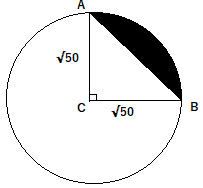mycollegehive
A chord 10 cm long is drawn in a circle of radius V50 cm. Find the area of minor segment
CBSE class-10 math areas-related-to-circles0A chord 10 cm long is drawn in a circle of radius V50 cm. Find the area of minor segment

24 viewsShareFollowUniversity of Benin Nigeria
01 August 2020School not set Nigeria
01 August 20200Length of cord = 2r sin(θ/2)

10 = 2√50 sin(θ/2)

divide both sides by  2√50

10/(2√50) = sin(θ/2)

√2 / 2 = sin(θ/2)

θ/2 = sin-1(√2 / 2 )

θ/2 = 45

θ = 90

Thus, the angle at the center of the circle is 90oThe shaded portion above AB represents the minor segment.

Area of minor segment = Area of sector ACB - Area of triangle ACB

Area of sector ACB = 1/2 (length of arc x radius)

length of arc = θ/360 x 2πr

Area of sector ACB = 1/2 (θ/360 x 2πr x r)

= 1/2 (θ/360 x 2πr2)

= θ/360 x πr2

r = √50

θ= 90

Thus, area of sector ACB = 90/360 x π x (√50)2

= 25π/2

but, π = 22/7

Area of sector ACB = 25/2 x 22/7

= 275/7

Area of triangle ACB = 1/2 base x height

= 1/2 x √50 x √50

= 25

Therefore, area of the segment = 275/7 - 25

= 100/7

= 14.285 cm2Share### Related Tags

CBSE

0 followers

56 questionsclass-10

0 followers

56 questionsmath

2 followers

985 questions